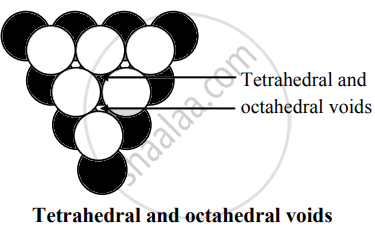# How are tetrahedral and octahedral voids formed? - Chemistry

How are tetrahedral and octahedral voids formed?

#### Solution

1. Two dimensional hexagonal close-packed layer has triangular voids which is formed by three spheres.
2. When the triangular voids of the first layer are covered by spheres of the next layer, tetrahedral voids are formed. A tetrahedral void is surrounded by four spheres.
3. The overlapping triangular voids from the two layers together form an octahedral void.
An octahedral void is surrounded by six spheres.
4. Thus, the depressions in which spheres of second layer rest are tetrahedral voids while the depressions in which no sphere rests are octahedral voids.Concept: Packing of Particles in Crystal Lattice
Is there an error in this question or solution?
Chapter 1: Solid State - Exercises [Page 27]

#### APPEARS IN

Balbharati Chemistry 12th Standard HSC for Maharashtra State Board
Chapter 1 Solid State
Exercises | Q 7 | Page 27
Share